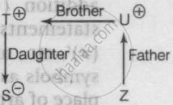# 'A + B' Means a is the Father of B, 'A - B' Means that a is the Wife of B, 'A X B' Means that a is the Brother of B and 'A ÷ B' Means that a is the Daughter of B. If 'S ÷ T X U + Z', Then Which of - Logical Reasoning

MCQ

'A + B' means A is the father of B, 'A - B' means that A is the wife of B, 'A x B' means that A is the brother of B and 'A ÷ B' means that A is the daughter of B. If 'S ÷ T x U + Z', then which of the following is true?

#### Options

• S is the daughter of U

• Z is the aunt of S

• S is the aunt of Z

• S and Z are cousins

#### Solution

S and Z are cousins
Explanation:
Given, S ÷ T × U + Z∴ S and Z are cousins is the true statement.

Concept: Blood Relationships (Entrance Exam)
Is there an error in this question or solution?